This vignette extends from the vignette (Basic maps) to demonstrate how `osmplotr` enables the graphical properties of OpenStreetMap objects to be modified according to user-provided data. Categorical data can be plotted by highlighting defined regions with different colours using `add_osm_groups`, while continuous data can be plotted with `add_osm_surface`.

``library (osmplotr)``

As in the first vignette, maps produced in this vignette contain data for a small portion of central London, U.K.

``bbox <- get_bbox (c (-0.13, 51.51, -0.11, 51.52))``

## 1. Categorical data: `add_osm_groups`

The function `add_osm_groups` enables spatially-defined groups to be plotted in different colours. The two primary arguments are `obj`, which defines the OSM structure to be used for plotting the regions, and `groups` which is a list of geometric coordinates defining the desired regions. An example of an `obj` is the Simple Features (`sf`) `data.frame` of building polygons downloaded in the first vignette with the following line

``dat_B <- extract_osm_objects (key = "building", bbox = bbox)``

These data may be obtained by simply combining the data provided with the package of residential and non-residential buildings to give all buildings as

``dat_B <- rbind (london\$dat_BNR, london\$dat_BR)``

The most direct way to define `groups` is through specifying coordinates of boundary points:

``````pts <- cbind (
c (-0.115, -0.125, -0.125, -0.115),
c (51.513, 51.513, 51.517, 51.517)
)

map <- osm_basemap (
bbox = bbox,
bg = "gray20"
)

dat_B,
groups = pts,
cols = "orange",
bg = "gray40"
)

print_osm_map (map)``````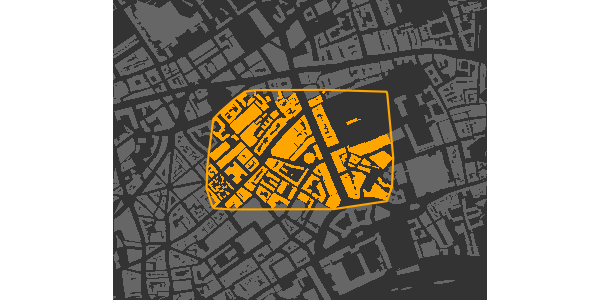Multiple groups can be defined by passing a list of multiple sets of point coordinates to the `groups` argument of `add_osm_groups`, and specifying corresponding colours.

``````pts2 <- cbind (
c (-0.111, -0.1145, -0.1145, -0.111),
c (51.517, 51.517, 51.519, 51.519)
)

map <- osm_basemap (
bbox = bbox,
bg = "gray20"
)

dat_B,
groups = list (pts, pts2),
cols = c ("orange", "tomato"),
bg = "gray40"
)

print_osm_map (map)``````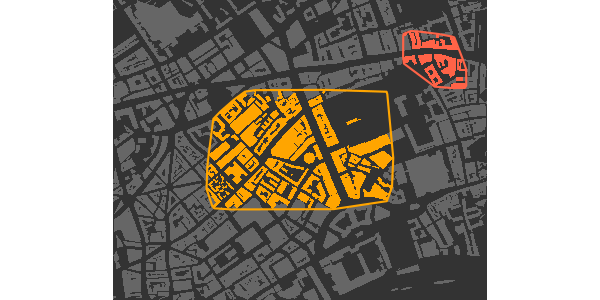The `bg` argument specifies the colour of any objects lying outside the boundaries of the specified groups. If this argument is not given, then all objects are assigned to the nearest group, so that the groups fill the entire map.

``````map <- osm_basemap (
bbox = bbox,
bg = "gray20"
)

dat_B,
groups = list (pts, pts2),
cols = c ("orange", "tomato")
)

print_osm_map (map)``````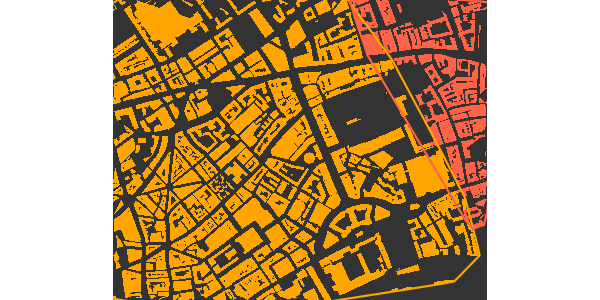Now that you’ve seen the general workflow of `osmplotr`, let’s repeat the previous code, but streamline it with `magrittr`’s `%>%` function. This allows us to pipe the functions together instead of re-assigning the `map` variable.

``````library (magrittr)

osm_basemap (
bbox = bbox,
bg = "gray20"
) %>%
groups = list (pts, pts2),
cols = c ("orange", "tomato")
) %>%
print_osm_map ()``````### 1.1 Hulls around groups

`add_osm_groups` includes the argument `make_hull` which specifies whether convex hulls should be fitted around the points defining the provided `groups`, or whether the `groups` already define their own boundaries (the default behaviour). If a point is added internal to the four points defining the first of the above groups, then the group boundary will connect to that point and create a concave shape.

``````pts <- rbind (pts, c (-0.12, 51.515))

osm_basemap (
bbox = bbox,
bg = "gray20"
) %>%
groups = pts,
cols = "orange",
bg = "gray40"
) %>%
print_osm_map ()``````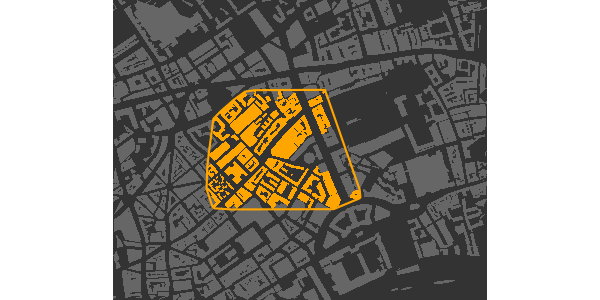The previous points started in the south-east and ended in the north-east, and thus the concave boundary extends in between the two easterly points. Setting `make_hull = TRUE` defines groups by the convex hulls surrounding them, which in this case would revert this map to the initial map with the group defined by a regular, convex perimeter.

### 1.2 Inclusive, exclusive, and bisected polygons

The highlighted regions of the previous maps are irregular because the default behaviour of `add_osm_groups` is to include within a group only those OSM objects which lie entirely within a group boundary. `add_osm_groups` has a `boundary` argument which defines whether objects should be assigned to groups inclusively (`boundary > 0`) or exclusively (`boundary < 0`), or whether they should be precisely bisected by a group boundary (`boundary = 0`). The previous maps illustrate the default option (`boundary = -1`), while the two other options produce the following maps.

``````osm_basemap (bbox = bbox, bg = "gray20") %>%
groups = list (pts, pts2),
make_hull = TRUE,
cols = c ("orange", "tomato"),
bg = "gray40",
boundary = 1
) %>%
print_osm_map ()``````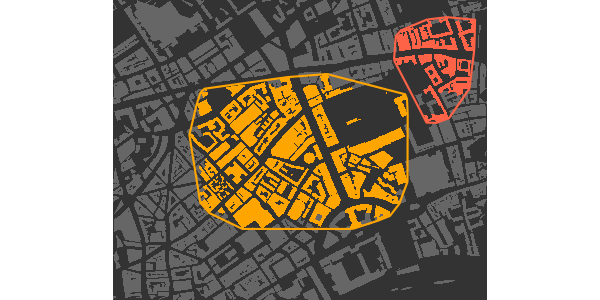The inclusive option (`boundary>0`) includes all objects which have any points lying within a boundary, meaning more objects are included resulting in larger regions than the previous default exclusive option. Precisely bisecting boundaries produces the following map.

``````osm_basemap (
bbox = bbox,
bg = "gray20"
) %>%
groups = list (pts, pts2),
make_hull = TRUE,
cols = c ("orange", "tomato"),
bg = "gray40",
boundary = 0
) %>%
print_osm_map ()``````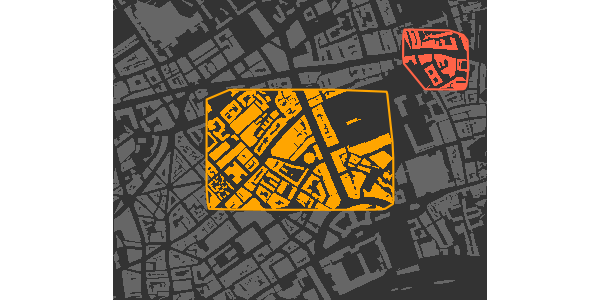The ability to combine different kinds of boundaries is particularly useful when highlighting areas which partially contain large polygons such as parks. The parks within the following maps were downloaded with

``dat_P <- extract_osm_objects (key = "park", bbox = bbox)``

(Noting that, as described in the first vignette, Basic maps, both `extract_osm_objects` and `make_osm_map` convert several common keys to appropriate `key-value` pairs, so

``osm_structures (structure = "park")``
``````##    structure     key value suffix      cols
## 1       park leisure  park      P #647864FF
## 2 background                         gray20``````

reveals that this `key` is actually converted to `key = "leisure"` and `value = "park"`.) These data are also provided with the package as `london\$dat_P`.

Plotting buildings inclusively within each group and overlaying parks bisected by the group boundaries produces the following map:

``````col_park_in <- rgb (50, 255, 50, maxColorValue = 255)
col_park_out <- rgb (50, 155, 50, maxColorValue = 255)

osm_basemap (
bbox = bbox,
bg = "gray20"
) %>%
groups = list (pts, pts2),
make_hull = TRUE,
cols = c ("orange", "tomato"),
bg = "gray40",
boundary = 0
) %>%
groups = list (pts, pts2),
cols = rep (col_park_in, 2),
bg = col_park_out,
boundary = 0
) %>%
print_osm_map ()``````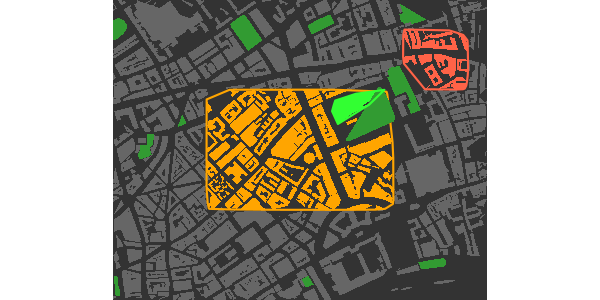Bisection divides single polygons to form one polygon of points lying within a given boundary and one polygon of points lying outside the boundary. The two resultant polygons are often separated by visible gaps between locations at which they are defined. Because the layers of a plot are progressively overlaid, such gaps can be avoided by initially plotting underlying layers using `add_osm_objects` prior to grouping objects:

``````map <- osm_basemap (
bbox = bbox,
bg = "gray20"
) %>%
col = col_park_out
) %>%
groups = list (pts, pts2),
cols = rep (col_park_in, 2),
bg = col_park_out,
boundary = 0
) %>%
groups = list (pts, pts2),
make_hull = TRUE,
cols = c ("orange", "tomato"),
bg = "gray40",
boundary = 0
)

map %>%
print_osm_map ()``````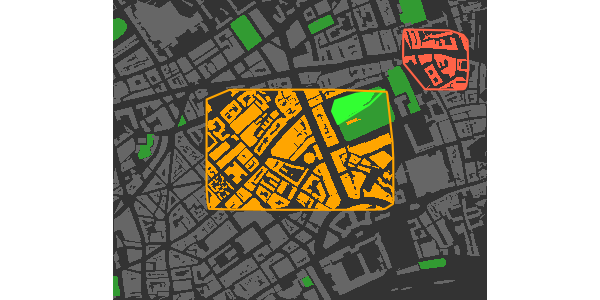Bisections with `boundary = 0` will only be as accurate as the underlying OSM data. This example was chosen to highlight that bisection may be inaccurate if actual OSM points do not lie near to a desired bisection line. The larger a map, the less visually evident are likely to be any such inaccuracies. Finally, note that the plot order was changed to allow the building within the park to be overlaid upon the grass surfaces. Plot order, whether controlled manually or with `make_osm_map`, may often have to be tweaked to appropriately visualise all objects.

The `boundary` argument has no effect if `bg` is not given, because in this case all objects will be assigned to a group and there will be no boundaries between groups and other, non-grouped objects.

### 1.3 Adjusting colours with `adjust_colours`

The `adjust_colours` function allows different groups to be highlighted with slightly different colours for different kinds of OSM objects. For example, the following code adds highways to the above map in slightly darkened versions of the highlight colours (using `boundary = 1`, so any highways with any points lying within the bounding box are included in the groups):

``````# create separate data for all highways and primary highways
dat_H <- rbind (london\$dat_H, london\$dat_HP)
dat_HP <- london\$dat_HP

# darken colours by aboud 20%
)

map %>%
groups = list (pts, pts2),
make_hull = TRUE,
),
boundary = 1, size = 2
) %>%
groups = list (pts, pts2),
make_hull = TRUE,
),
boundary = 1,
size = 1
) %>%
print_osm_map ()``````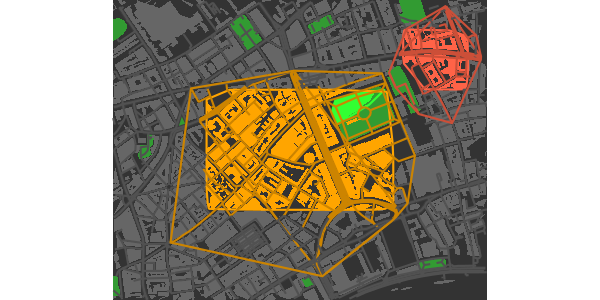And of course `adjust_colours ("gray40", adj = -0.2)` is nothing other than “gray32”, and `adj = -0.4` gives “gray24”.

### 1.4 Dark-on-Light Highlights

A particularly effective way to highlight single regions within a map is through using dark colours upon otherwise light coloured maps.

``````osm_basemap (bbox = bbox, bg = "gray95") %>%
groups = pts,
cols = "gray40",
bg = "gray85",
boundary = 1
) %>%
groups = pts,
cols = "gray20",
bg = "gray70",
boundary = 0
) %>%
groups = pts,
cols = "gray10",
bg = "white",
boundary = 0,
size = 1
) %>%
print_osm_map ()``````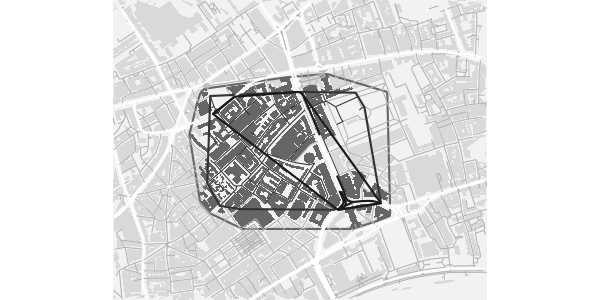### 1.5 Visualising clustering data

One of the most likely uses of `add_osm_groups` is to visualise statistical clusters. Clustering algorithms will generally produce membership lists which may be mapped onto spatial locations. Each cluster can be defined as a matrix of points in a single list of `groups`. A general approach is illustrated here with `groups` defined by single, randomly generated points.

``````set.seed (2)
ngroups <- 12
x <- bbox [1, 1] + runif (ngroups) * diff (bbox [1, ])
y <- bbox [2, 1] + runif (ngroups) * diff (bbox [2, ])
groups <- as.list (data.frame (t (cbind (x, y))))``````

(The last line just transforms each row of the matrix into a list item.) Having generated the points, a map of corresponding clusters can be generated by the following simple code.

``````osm_basemap (
bbox = bbox,
bg = "gray95"
) %>%
groups = groups,
cols = rainbow (length (groups))
) %>%
print_osm_map ()``````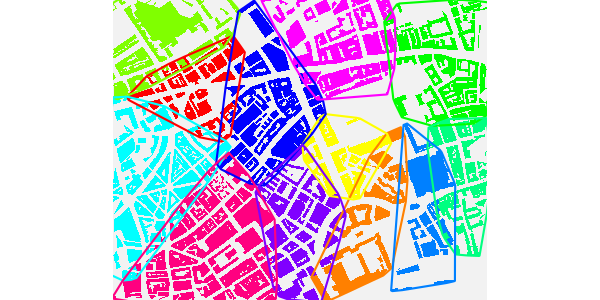Although individual groups will generally be defined by collections of multiple points, this example illustrates that they can also be defined by single points. In such cases, the `bg` option should of course be absent, so that all remaining points are allocated to the nearest groups.

This map also illustrates the kind of visual mess that may arise in attempts to specify colours, particularly because the sequence of colours passed to `add_osm_groups` will generally not map on to any particular spatial order, so even if a pleasing colour scheme is submitted, the results may still be less than desirable. Although it may be possible to devise pleasing schemes for small numbers of groups, manually defined colour schemes are likely to become impractical for larger numbers of groups.

``````osm_basemap (
bbox = bbox,
bg = "gray95"
) %>%
groups = groups,
border_width = 2,
cols = heat.colors (length (groups))
) %>%
print_osm_map ()``````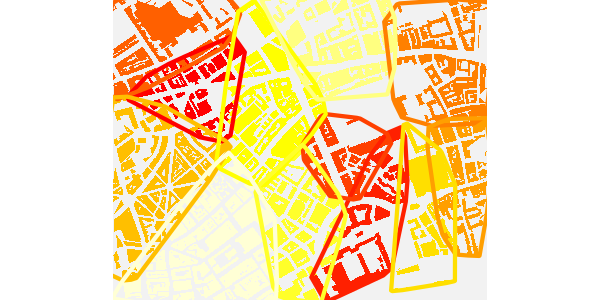Note the submitting any positive values to the additional `border_width` argument causes `add_osm_groups` to drawn convex hull borders around the different groups. Even this is not sufficient, however, to render the result particularly visually pleasing or intelligible. To overcome this, `add_osm_groups` includes an option described in the following section to generate spatially sensible colour schemes for colouring distinct groups.

### 1.6 The Colour Matrix: Colouring Several Regions

An additional argument which may be passed to `add_osm_groups` is `colmat`, an abbreviation of ‘colour matrix’. If set to true (the default is `FALSE`), group colours are specified by the function `colour_mat`. This function takes a vector of four or more colours as input, wraps them around the four corners of a rectangular grid, and spatially interpolates a chromatically regular grid between these corners. To visual different schemes, it has a `plot` argument:

``cmat <- colour_mat (rainbow (4), plot = TRUE)``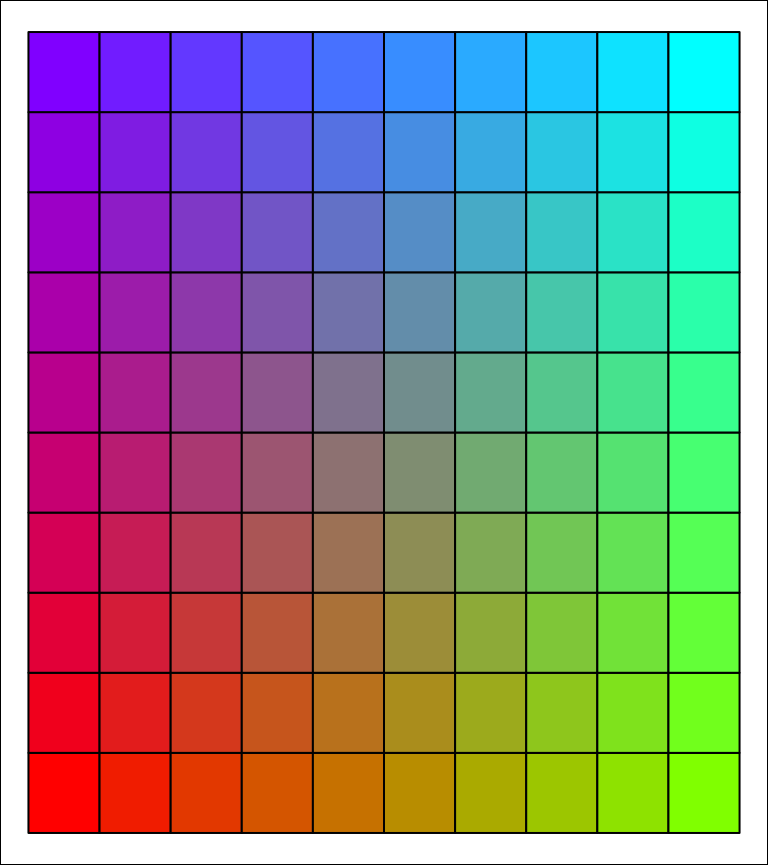This grid illustrates the default colours, `rainbow (4)`. The two-dimensional colour field produced by `colour_mat` may also be rotated by a specified number of degrees using the `rotate` argument.

``cmat <- colour_mat (rainbow (4), n = c (4, 8), rotate = 90, plot = TRUE)``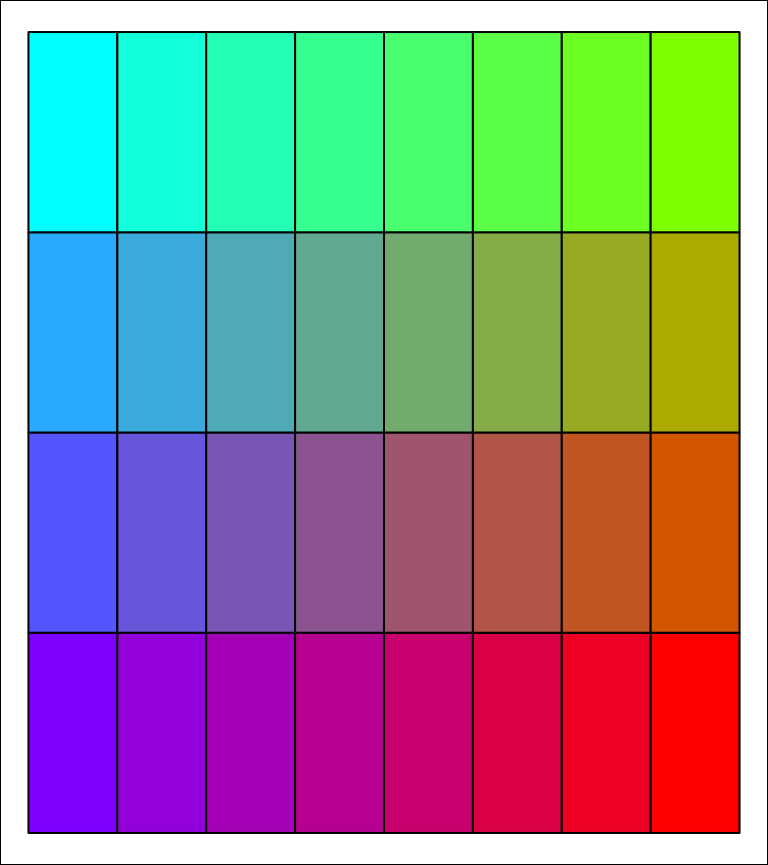This example also illustrates that the size of colour matrices may also be arbitrarily specified. Using the `colmat` option in `add_osm_groups` enables the previous maps to be redrawn like this:

``````osm_basemap (
bbox = bbox,
bg = "gray95"
) %>%
groups = groups,
border_width = 2,
colmat = TRUE,
cols = c ("red", "green", "yellow", "blue"),
rotate = 180
) %>%
print_osm_map ()``````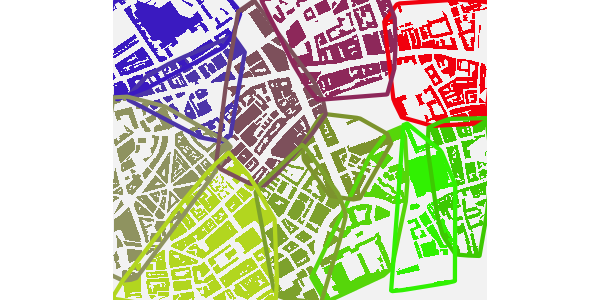Note both that when `add_osm_groups` is called with `colmat = TRUE`, then `cols` need only be of length 4, to specify the four corners of the colour matrix, and also that the `rotate` argument can be submitted to `add_osm_groups` and passed on to `colour_mat`.

### 1.7 Bounding areas within named highways

As explained in the first vignette, Basic maps, the function `connect_highways` takes a list of OSM highway names and a bounding box, and returns the boundary of a polygon encircling the named highways. This can be used to highlight selected regions simply by naming the highways which encircle them, producing maps which look like this:

``````highways <- c (
"Monmouth.St", "Short.?s.Gardens", "Endell.St", "Long.Acre",
"Upper.Saint.Martin"
)
highways1 <- connect_highways (highways = highways, bbox = bbox)
highways <- c ("Endell.St", "High.Holborn", "Drury.Lane", "Long.Acre")
highways2 <- connect_highways (highways = highways, bbox = bbox)
highways <- c ("Drury.Lane", "High.Holborn", "Kingsway", "Great.Queen.St")
highways3 <- connect_highways (highways = highways, bbox = bbox)``````

Note the use of the regex character `?` in the first list of highway names, denoting the previous character as optional. This is necessary here because there are OSM sections named both “Shorts Gardens” and “Short’s Gardens”.

``class (highways1)``
``##  "matrix" "array"``
``nrow (highways1)``
``##  41``
``nrow (highways2)``
``##  33``
``nrow (highways3)``
``##  53``

`connect_highways` returns a list of `SpatialPoints` representing the shortest path that sequentially connects all of the listed highways. (Connecting all listed highways may not necessarily be possible, in which case warnings will be issued. As described in the first vignette, Basic maps, `connect_highways` also has a `plot` option allowing problematic cases to be visually inspected and hopefully corrected.)

These lists of highway coordinates can then be used to highlight the areas they encircle. First group the highways and establish a colour scheme for the map:

``````groups <- list (highways1, highways2, highways3)
cols_B <- c ("red", "orange", "tomato") # for the 3 groups
bg_B <- "gray40"
bg_H <- "gray60"``````

And then plot the map.

``````osm_basemap (bbox = bbox, bg = "gray20") %>%
col = col_park_out
) %>%
groups = groups,
boundary = 1,
bg = bg_B,
cols = cols_B
) %>%
groups = groups,
boundary = 1,
bg = bg_H,
cols = cols_H
) %>%
groups = groups,
boundary = 0,
cols = cols_H,
bg = bg_H,
size = 1
) %>%
print_osm_map ()``````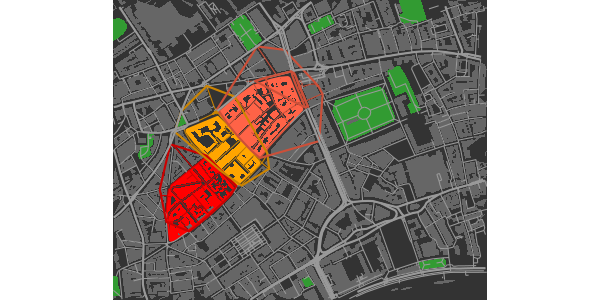These encircling highways are included in the `london` data provided with `osmplotr`.

## 2. Continuous data: `add_osm_surface`

The `add_osm_surface` function enables a continuous data surface to be overlaid on a map. User-provided data is spatially interpolated across a map region and OSM items coloured according to a specified continuous colour gradient. The data must be provided as a data frame with three columns, ‘(x,y,z)’, where ‘(x,y)’ are the coordinates of points at which data are given, and ‘z’ are the values to be spatially interpolated across the map.

A simple data frame can be constructed as

``````n <- 5
x <- seq (bbox [1, 1], bbox [1, 2], length.out = n)
y <- seq (bbox [2, 1], bbox [2, 2], length.out = n)
dat <- data.frame (
x = as.vector (array (x, dim = c (n, n))),
y = as.vector (t (array (y, dim = c (n, n)))),
z = x * y
)
``````##        x       y         z
## 1 -0.130 51.5100 -6.696300
## 2 -0.125 51.5100 -6.439063
## 3 -0.120 51.5100 -6.181800
## 4 -0.115 51.5100 -5.924512
## 5 -0.110 51.5100 -5.667200
## 6 -0.130 51.5125 -6.696300``````

And then passed to `add_osm_surface`

``````osm_basemap (
bbox = bbox,
bg = "gray20"
) %>%
dat = dat,
cols = heat.colors (30)
) %>%
print_osm_map ()``````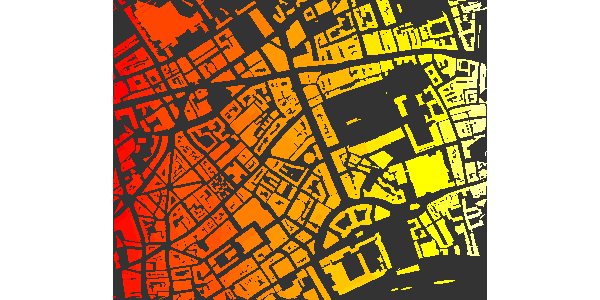At present, `add_osm_surface` generates an warning if it is applied more than once to any one kind of `Spatial` object (polygons or lines), as illustrated in the following code (in which both `dat_H` and `dat_HP` are of class `SpatialLinesDataFrame`:

``````osm_basemap (bbox = bbox, bg = "gray20") %>%
dat = dat,
cols = heat.colors (30)
) %>%
dat = dat,
cols = heat.colors (30)
)``````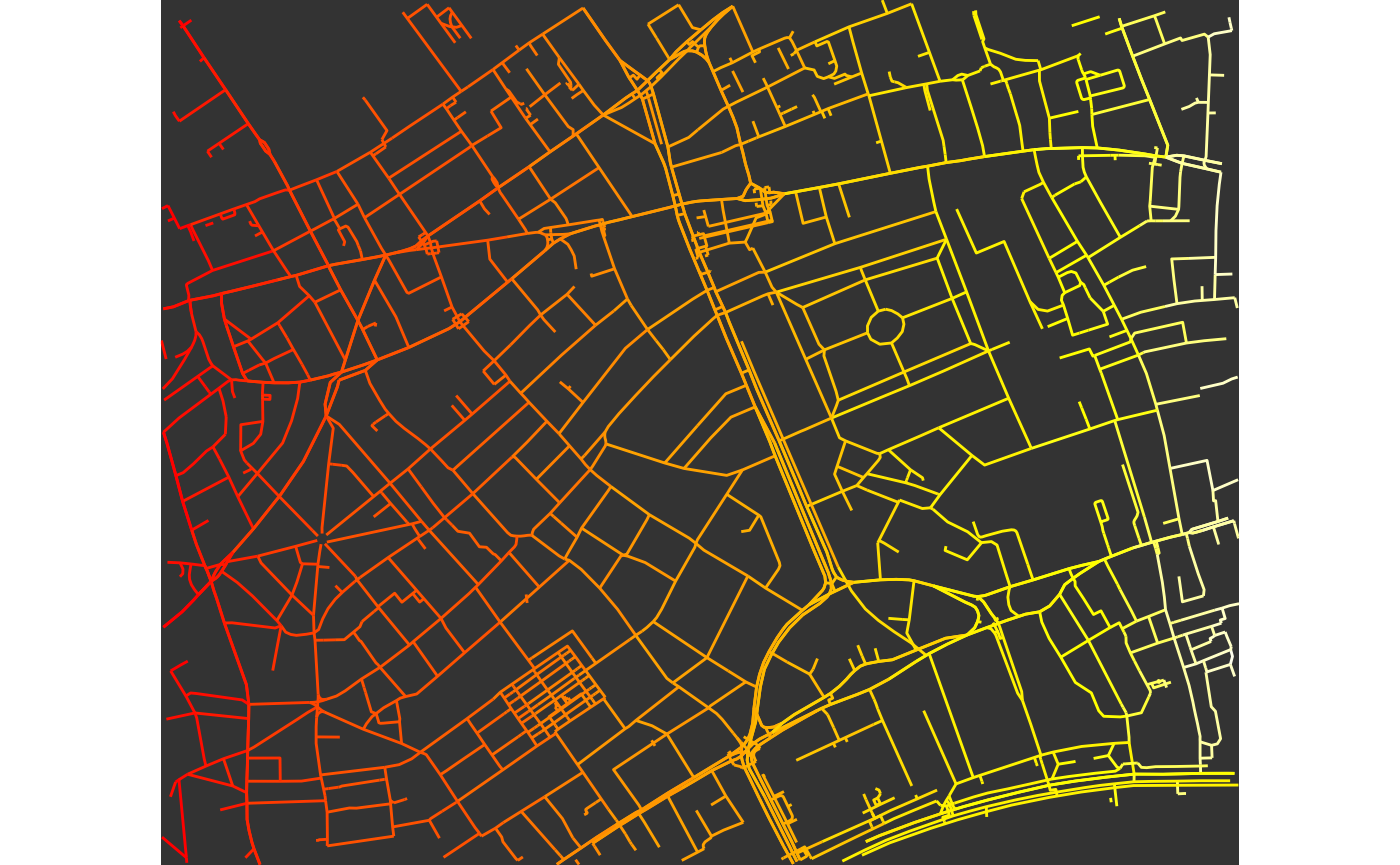This is because `add_osm_surface` creates new `ggplot2` aesthetic schemes for each kind of object, and these schemes are not intended to be modified or replaced within a single plot. The above map may still be printed, but the warning means that the last provided colour scheme will be applied to all objects of that class. This means that `osmplotr` can only overlay two distinct colour schemes: one for all objects of class `SpatialLines`, and a potentially different one for all objects of class `SpatialPolygons`.

Of course, any number of additional objects may be overlaid with `add_osm_objects`, for example,

``````cols_adj <- adjust_colours (heat.colors (30), -0.2)

map <- osm_basemap (
bbox = bbox,
bg = "gray20"
) %>%
dat = dat,
cols = heat.colors (30)
) %>%
dat = dat,
size = 1.5
) %>%
col = rgb (0.1, 0.3, 0.1)
) %>%
col = "gray60"
)

map %>%
print_osm_map ()``````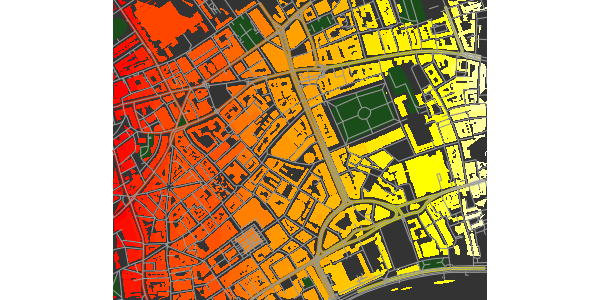### 2.1 Colourbar legends for data surfaces

A colourbar legend for the surface may be added with `add_colourbar`. As with `add_axes`, this function is provided separately to allow colourbars to be overlaid only after all desired map items have been added. The only parameters required for `add_colourbar` are the limits of the data (`zlims`) and the colours (along with the `map`, a modified version of which is returned).

``````map %>%
cols = terrain.colors (100),
zlims = range (dat\$z)
) %>%
print_osm_map ()``````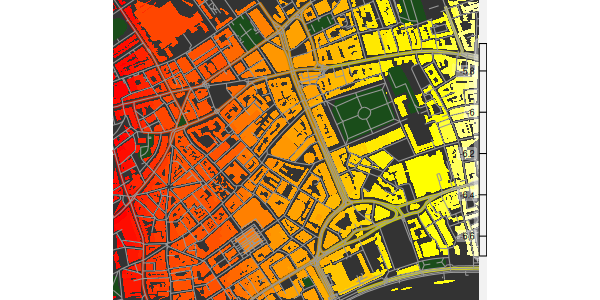Note that the colours submitted to `add_colourbar` need not be the same as those used to plot the surface. (Although using different colours is rarely likely to be useful.) As for `add_axes`, and explained in the first vignette, Basic maps, the transparency of the boxes surrounding the elements of the colourbar may be controlled by specifying the value of `alpha`. Both alignment and position may also be adjusted, as illustrated in this example.

``````cols_adj <- adjust_colours (heat.colors (30), -0.2)

osm_basemap (
bbox = bbox,
bg = "gray20"
) %>%
dat = dat,
cols = heat.colors (30)
) %>%
dat = dat,
size = 1.5
) %>%
cols = heat.colors (100),
zlims = range (dat\$z),
alpha = 0.9,
vertical = FALSE,
barwidth = c (0.1, 0.12),
barlength = c (0.5, 0.9),
text_col = "blue",
fontsize = 5,
fontface = 3,
fontfamily = "Times"
) %>%
colour = "blue",
fontsize = 5,
fontface = 3,
fontfamily = "Times"
) %>%
print_osm_map ()``````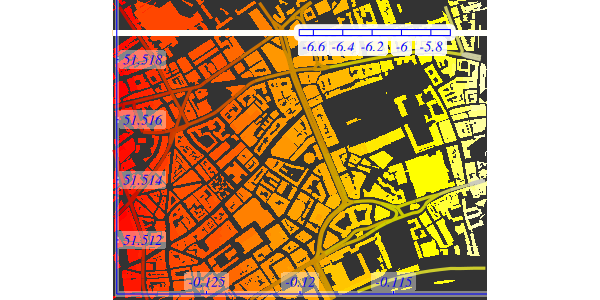Both `barwidth` and `barlength` can be specified in terms of one or two numbers. A single value for `barwidth` determines its relative width (0-1) from the border (the right side if `vertical = TRUE` or the top if `vertical = FALSE`), while two values determine the relative start and end positions of the sides of the bar. A single value for `barlength` produces a bar of the given length centred in the middle of the map, while two values determine its respective upper and lower points (for `vertical = TRUE`) or left and right points (for `vertical = FALSE`).

This example also demonstrates how colours, sizes, and other font characteristics of text labels can be specified (with `text_col` determining the colour of all elements of the colourbar other than the gradient itself). Finally, as for `add_axes`, the text labels of colourbars are not currently able to be rotated because `ggplot2` does not permit rotation for the `geom_label` function used to produce these labels.

### 2.1 Surfaces and data perimeters

It may often be that user-provided data only extend across a portion of a map, leaving a perimeter beyond the data boundary for which interpolation should not be applied. `add_osm_surface` has a `bg` parameter specifying a background colour for objects beyond the perimeter of the data surface. Passing this parameter to `add_osm_surface` causes objects beyond the data perimeter to be coloured within this ‘background’ colour.

To illustrate, trim the above data to within a circular range of the centre of the map.

``````d <- sqrt ((dat\$x - mean (dat\$x))^2 + (dat\$y - mean (dat\$y))^2)
range (d)``````
``##  0.00000000 0.01118034``

Remove from `dat` all rows translating to `d>0.01`:

``````dat <- dat [which (d < 0.01), ]

osm_basemap (
bbox = bbox,
bg = "gray20"
) %>%
dat = dat,
cols = heat.colors (30),
bg = "gray40"
) %>%
dat = dat,
size = c (1.5, 0.5),
bg = "gray70"
) %>%
print_osm_map ()``````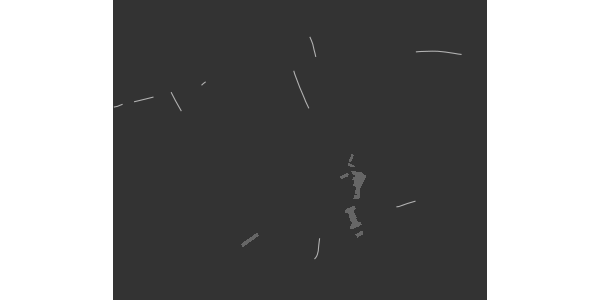(The perimeter is irregular because of the positions of the points in `dat`.)

### 2.3 Further control of surface appearance

The final `add_osm_surface` call in the above code (for `dat_HP`) illustrates additional parameters that may be passed for further control of map appearance. In this case, the two `size` parameters control the size of the lines within the data surface and beyond its perimeter. Single values may also be passed, in which case they determine the width of lines in both cases. One or two `shape` parameters may also be passed, with these also determining the shapes of `SpatialPoints`, as illustrated in the next example, which overlays trees on the map.

Both lines and points use the same `ggplot2` colour gradient, and so adding the second of these again generates an error and means that the actual colour scheme will be determined by the final call to add either lines or points.

``dat_T <- extract_osm_objects (key = "tree", bbox = bbox)``
``````osm_basemap (
bbox = bbox,
bg = "gray20"
) %>%
dat = dat,
cols = terrain.colors (30),
size = c (1.5, 0.5),
bg = "gray70"
) %>%
dat = dat,
cols = terrain.colors (30),
size = c (1, 0.5),
bg = "gray70"
) %>%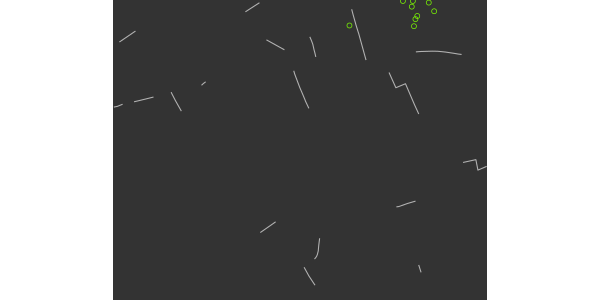The first two colour specifications (`terrain.colors`) have been ignored, and all added items are coloured according to the final value of `heat.colors (30)`. Other aspects such as line sizes and point shapes are nevertheless respected.